Aishwarya Singh — Published On August 9, 2018 and Last Modified On July 21st, 2022

## Introduction

Have you ever tried working with a large dataset on a 4GB RAM machine? It starts heating up while doing simplest of machine learning tasks? This is a common problem data scientists face when working with restricted computational resources.

When I started my data science journey using python, I almost immediately realized that the existing libraries have certain limitations when it comes to handling large datasets. Pandas and Numpy are great libraries but they are not always computationally efficient, especially when there are GBs of data to manipulate. So what can you do to get around this obstacle?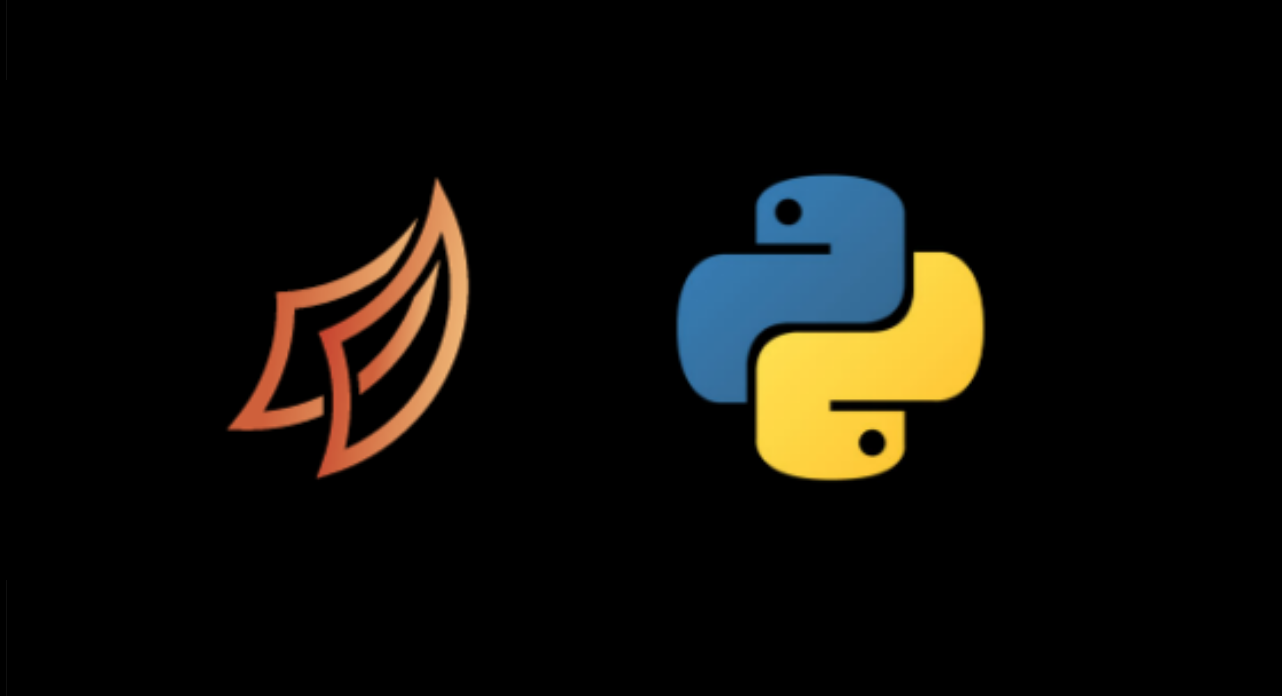This is where Dask weaves its magic! It works with Pandas dataframes and Numpy data structures to help you perform data wrangling and model building using large datasets on not-so-powerful machines. Once you start using Dask, you won’t look back.

In this article, we will look at what Dask is, how it works, and how you can use it for working on large datasets. We will also take up a dataset and put Dask to good use. Let’s begin!

1. A Simple Example to Understand Dask
2. Challenges with common Data Science Python libraries
6. Working on a dataset

## 1. A Simple Example to Understand Dask

Let me illustrate these aforementioned limitations with a simple example. Suppose you have 4 balls (of different colors) and you are asked to separate them within an hour (based on the color) into different buckets.What if you are given a hundred balls and you have to separate them in an hour’s time? That would be a tedious task but still sounds feasible. Imagine you are given a thousand balls and an hour to separate them into buckets. It is impossible for an individual to complete the task within the given time (in this case, the data is huge and the resources are limited). How would you accomplish this?

The best bet would be to ask a few other people for help. You can call 9 other friends, give each of them 100 balls and ask them to separate these based on the color. In this case, 10 people are simultaneously working on the assigned task and together would be able to complete it faster than a single person would have (here you had a huge amount of data which you distributed among a bunch of people).

Currently we use common libraries like pandas, numpy and scikit-learn for data preprocessing and model building. These libraries are not scalable and work on a single CPU. Dask however can scale up to a cluster of machines. To sum up, pandas and numpy are like the individual trying to sort the balls alone, while the group of people working together represent Dask.

## 2. Challenges with Common Data Science Python Libraries (Numpy, Pandas, Sklearn)

Python is one of the most popular programming languages today and is widely used by data scientists and analysts across the globe. There are common python libraries (numpy, pandas, sklearn) for performing data science tasks and these are easy to understand and implement.

But when it comes to working with large datasets using these python libraries, the run time can become very high due to memory constraints. These libraries usually work well if the dataset fits into the existing RAM. But if we are given a large dataset to analyze (like 8/16/32  GB or beyond), it would be difficult to process and model it. Unfortunately, these popular libraries were not designed to scale beyond a single machine. It is like asking a single person to separate a thousand balls in a limited time frame, it’s quite unfair to ask!

What should one do when faced with a dataset larger than what a single machine can process? This is where Dask comes into the picture. It is a python library that can handle moderately large datasets on a single CPU by using multiple cores of machines or on a cluster of machines (distributed computing).

If you are familiar with pandas and numpy, you will find working with Dask fairly easy. Dask is popularly known as a ‘parallel computing’ python library that has been designed to run across multiple systems. Your next question would understandably be – what is parallel computing?

As in our example of separating the balls, 10 people doing the job simultaneously can be considered analogous to parallel computation. In technical terms, parallel computation is performing multiple tasks (or computations) simultaneously, using more than one resource.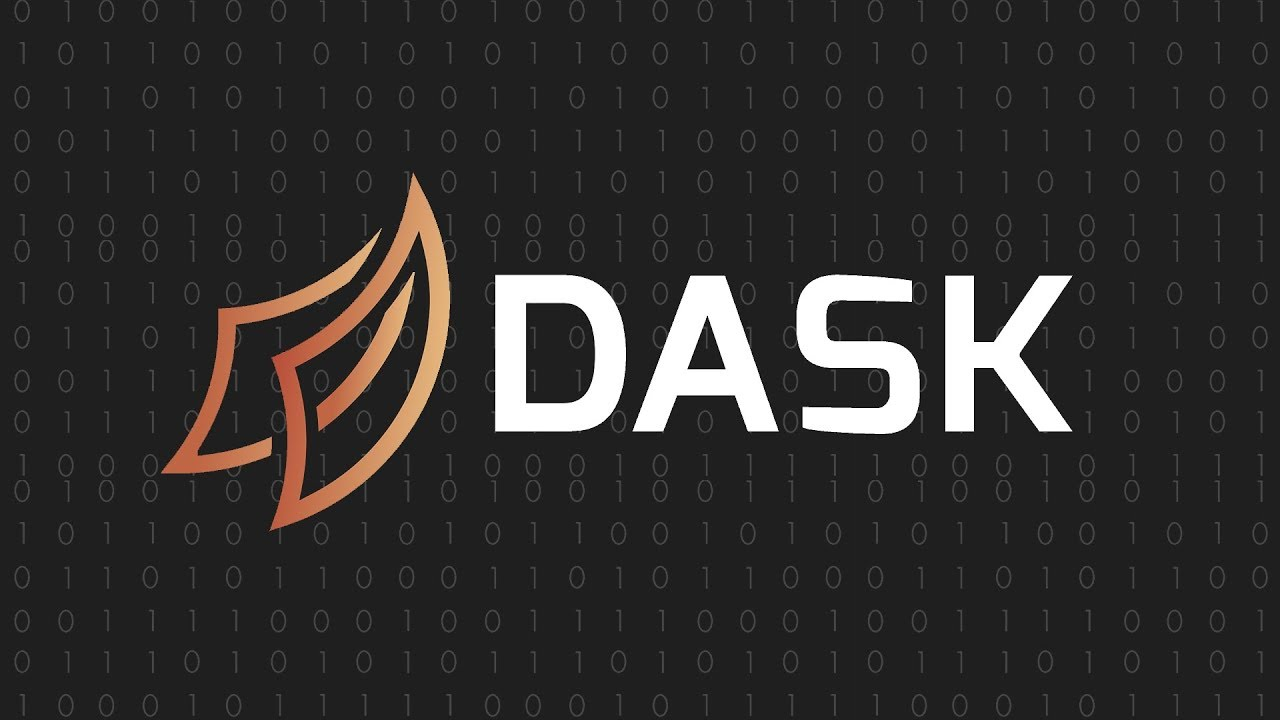Dask can efficiently perform parallel computations on a single machine using multi-core CPUs. For example, if you have a quad core processor, Dask can effectively use all 4 cores of your system simultaneously for processing. In order to use lesser memory during computations, Dask stores the complete data on the disk, and uses chunks of data (smaller parts, rather than the whole data) from the disk for processing. During the processing, the intermediate values generated (if any) are discarded as soon as possible, to save the memory consumption.

In summary, Dask can run on a cluster of machines to process data efficiently as it uses all the cores of the connected machines. One interesting fact here is that it is not necessary that all machines should have the same number of cores. If one system has 2 cores while the other has 4 cores, Dask can handle these variations internally.

Dask supports the Pandas dataframe and Numpy array data structures to analyze large datasets. Basically, Dask lets you scale pandas and numpy with minimum changes in your code format. How great is that?

Before we go ahead and explore the various functionalities provided by Dask, we need to setup our system first. Dask can be installed with conda, with pip, or directly from the source. This section explores all three options.

### 4.1 Using conda

Dask is installed in Anaconda by default. You can update it using the following command:

```conda install dask
```

### 4.2 Using pip

To install Dask using pip, simply use the below code in your command prompt/terminal window:

`pip install “dask[complete]”`

### 4.3 From source

1. Clone the git repository

```git clone https://github.com/dask/dask.git
python setup.py install```

2. Use pip to install all dependencies

`pip install -e “.[complete]”`

Now that we are familiar with Dask and have set up our system, let us talk about the Dask interface before we jump over to the python code. Dask provides several user interfaces, each having a different set of parallel algorithms for distributed computing. For data science practitioners looking for scaling numpy, pandas and scikit-learn, following are the important user interfaces:

• Arrays: parallel Numpy
• Dataframes: parallel Pandas
• Machine Learning: parallel Scikit-Learn

A large numpy array is divided into smaller arrays which, when grouped together, form the Dask array. In simple words, Dask arrays are distributed numpy arrays! Every operation on a Dask array triggers operations on the smaller numpy arrays, each using a core on the machine. Thus all available cores are used simultaneously enabling computations on arrays which are larger than the memory size.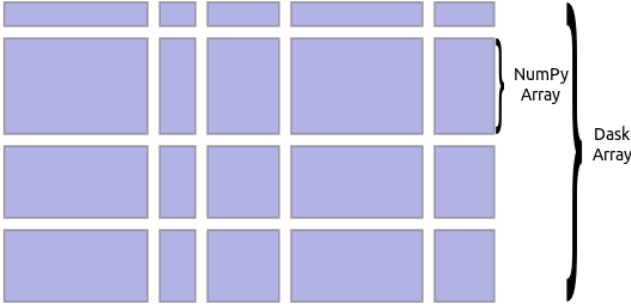As you can see, a number of numpy arrays are arranged into grids to form a Dask array. While creating a Dask array, you can specify the chunk size which defines the size of the numpy arrays. For instance, if you have 10 values in an array and you give the chunk size as 5, it will return 2 numpy arrays with 5 values each.

In summary, below are a few important features of Dask arrays below:

1. Parallel: Dask arrays use all the cores of the system
2. Larger-than-memory: Enables working on datasets that are larger than the memory available on the system (happens too often for me!). This is done by breaking the array into many small arrays and then performing the required operation
3. Blocked Algorithms: Perform large computations by performing many smaller computations. This is equivalent to sorting 1000 balls (large computation) by dividing it into 10 sets and sorting 100 balls (smaller computation)

We will now have a look at some simple cases for creating arrays using Dask.

1. Create a random array using Dask array

Python Code:

As you can see here, I had 11 values in the array and I used the chunk size as 5.  This distributed my array into three chunks, where the first and second blocks have 5 values each and the third one has 1 value.

1. Convert a numpy array to Dask array
```import numpy as np
x = np.arange(10)
y = da.from_array(x, chunks=5)
y.compute() #results in a dask array

array([0, 1, 2, 3, 4, 5, 6, 7, 8, 9])```

Dask arrays support most of the numpy functions. For instance, you can use .sum() or .mean(), as we will do now.

1. Calculating mean of the first 100 numbers
```import numpy as np

x = np.arange(1000)  #arange is used to create array on values from 0 to 1000
y = da.from_array(x, chunks=(100))  #converting numpy array to dask array

y.mean().compute()  #computing mean of the array

499.5```

Here, we simply converted our numpy array into a Dask array and used .mean() to do the operation.

In all the above codes, you must have noticed that we used .compute() to get the results. This is because when we simply use dask_array.mean(), Dask builds a graph of tasks to be executed. To get the final result, we use the .compute() function which triggers the actual computations.

We saw that multiple numpy arrays are grouped together to form a Dask array. Similar to a Dask array, a Dask dataframe consists of multiple smaller pandas dataframes. A large pandas dataframe splits row-wise to form multiple smaller dataframes. These smaller dataframes are present on a disk of a single machine, or multiple machines (thus allowing to store datasets of size larger than the memory). Each computation on a Dask dataframe parallelizes operations on the existing pandas dataframes.

Below is an image that represents the structure of a Dask dataframe: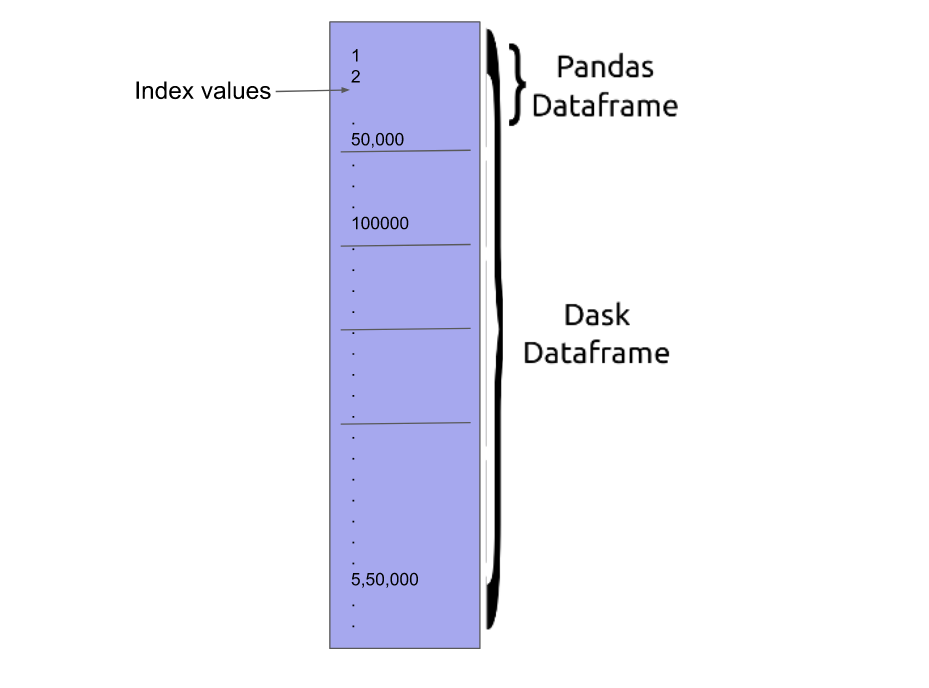The APIs offered by the Dask dataframe are very similar to that of the pandas dataframe.

```#reading the file using pandas
import pandas as pd

CPU times: user 485 ms, sys: 55.9 ms, total: 541 ms
Wall time: 506 ms```

```#reading the file using dask

CPU times: user 32.3 ms, sys: 3.63 ms, total: 35.9 ms
Wall time: 18 ms```

The Black Friday dataset used here has 5,50,068 rows. On using Dask, the read time reduced more than ten times as compared to using pandas!

1. Finding value count for a particular column
``` df.Gender.Value_counts().compute()

M    414259
F    135809
Name: Gender, dtype: int64```
1. Using groupby on the Dask dataframe
```#finding maximum value of purchase for both genders

df.groupby(df.Gender).Purchase.max().compute()

Gender
F    23959
M    23961
Name: Purchase, dtype: int64```

Dask ML provides scalable machine learning algorithms in python which are compatible with scikit-learn. Let us first understand how scikit-learn handles the computations and then we will look at how Dask performs these operations differently.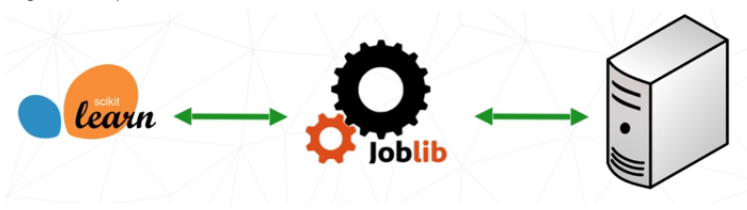A user can perform parallel computing using scikit-learn (on a single machine) by setting the parameter njobs = -1. Scikit-learn uses Joblib to perform these parallel computations. Joblib is a library in python that provides support for parallelization. When you call the .fit() function, based on the tasks to be performed (whether it is a hyperparameter search or fitting a model), Joblib distributes the task over the available cores. To understand Joblib in detail, you can have a look at this documentation.

Even though parallel computations can be performed using scikit-learn, it cannot be scaled to multiple machines. On the other hand, Dask works well on a single machine and can also be scaled up to a cluster of machines.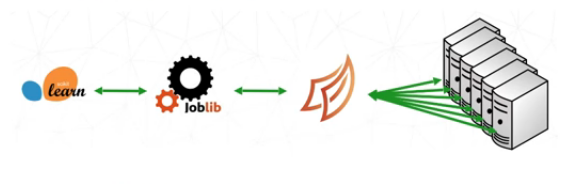Dask has a central task scheduler and a set of workers. The scheduler assigns tasks to the workers. Each worker is assigned a number of cores on which it can perform computations. The workers provide two functions:

• compute tasks as assigned by the scheduler
• serve results to other workers on demand

Below is an example that explains how a conversation between a scheduler and workers looks like (this has been given by one of the developers of Dask, Matthew Rocklin):

The central task scheduler sends jobs (python functions) to lots of worker processes, either on the same machine or on a cluster:

• Worker A, please compute x = f(1), Worker B please compute y = g(2)
• Worker A, when g(2) is done please get y from Worker B and compute z = h(x, y)

### 5.3.1 ML models

Dask-ML provides scalable machine learning in python which we will discuss in this section. Implementation for the same will be covered in section 6. Let us first get our systems ready. Below are the installation steps for Dask-ML.

```# Install with conda

# Install with pip

1. Parallelize Scikit-Learn Directly

As we have seen previously, sklearn provides parallel computing (on a single CPU) using Joblib. In order to parallelize multiple sklearn estimators, you can directly use Dask by adding a few lines of code (without having to make modifications in the existing code).

The first step is to import client from dask.distributed. This command will create a local scheduler and worker on your machine.

```from dask.distributed import Client
client = Client() # start a local Dask client```

The next step will be to instantiate dask joblib in the backend. You need to import parallel_backend from sklearn joblib like I have shown below.

```import dask_ml.joblib
from sklearn.externals.joblib import parallel_backend
# Your normal scikit-learn code here
from sklearn.ensemble import RandomForestClassifier
model = RandomForestClassifier()```

2. Reimplement Algorithms with Dask Array

For simple machine learning algorithms which use Numpy arrays, Dask ML re-implements these algorithms. Dask replaces numpy arrays with Dask arrays to achieve scalable algorithms. This has been implemented for:

• Linear models (linear regression, logistic regression, poisson regression)
• Pre-processing (scalers , transforms)
• Clustering (k-means, spectral clustering)

A.  Linear model example

```from dask_ml.linear_model import LogisticRegression

model = LogisticRegression()
model.fit(data, labels)```

B. Pre-processing example

```from dask_ml.preprocessing import OneHotEncoder

encoder = OneHotEncoder(sparse=True)
result = encoder.fit(data)
```

C. Clustering example

```from dask_ml.cluster import KMeans
model = KMeans()
model.fit(data)```

Hyperparameter tuning is an important step in model building and can greatly affect the performance of your model. Machine learning models have multiple hyperparameters and it is not easy to figure out which parameter would work best for a particular case. Performing this task manually is generally a tedious process. In order to simplify the process, sklearn provides Gridsearch for hyperparameter tuning. The user is required to give the values for parameters and Gridsearch gives you the best combination of these parameters.

Consider an example where you choose a random forest technique to fit the dataset. Your model has three important tunable parameters – parameter 1, parameter 2 and parameter 3. You set the values for these parameters as:

Parameter 1 – Bootstrap = True

Parameter 2 – max_depth – [8, 9]

Parameter 3 – n_estimators : [50, 100 , 200]

sklearn Gridsearch : For each combination of the parameters, sklearn Gridsearch executes the tasks, sometimes ending up repeating a single task multiple times. As you can see from the below graph, this is not exactly the most efficient method: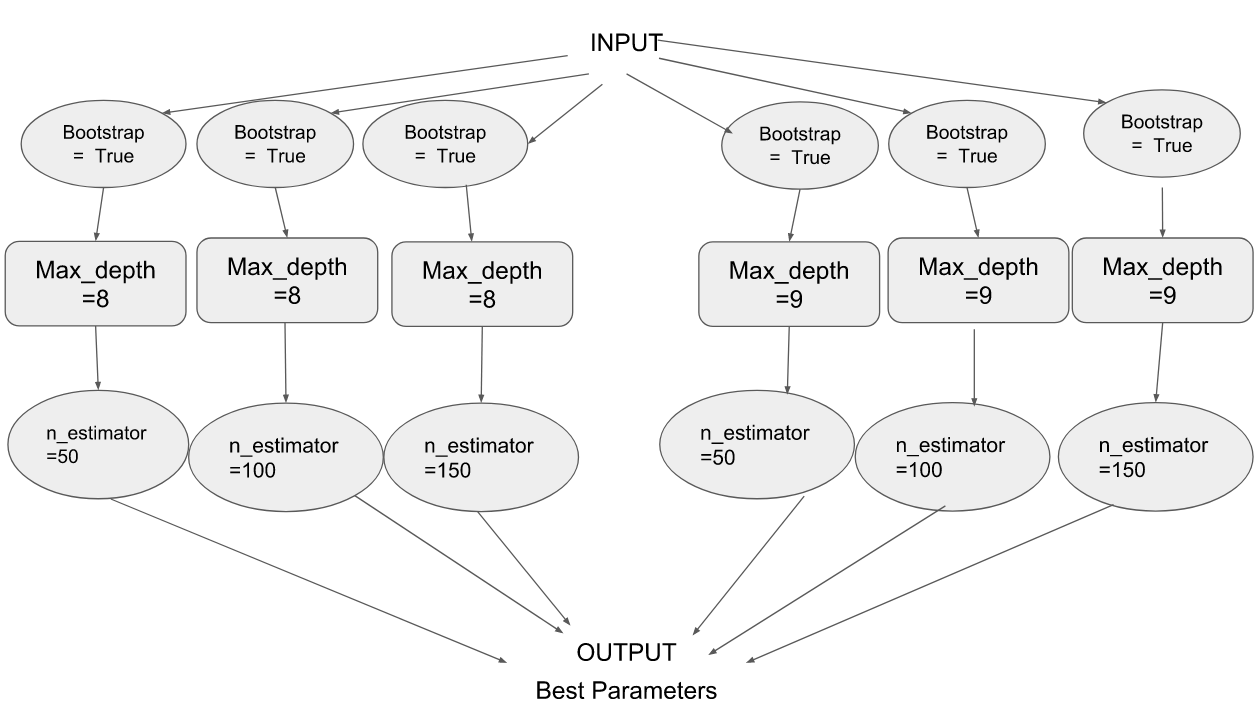Dask-Search CV: Parallel to Gridsearch CV in sklearn, Dask provides a library called Dask-search CV (Dask-search CV is now included in Dask ML). It merges steps so that there are less repetitions. Below are the installation steps for Dask-search.

```# Install with conda

# Install with pip

The following graph explains the working of Dask-Search CV: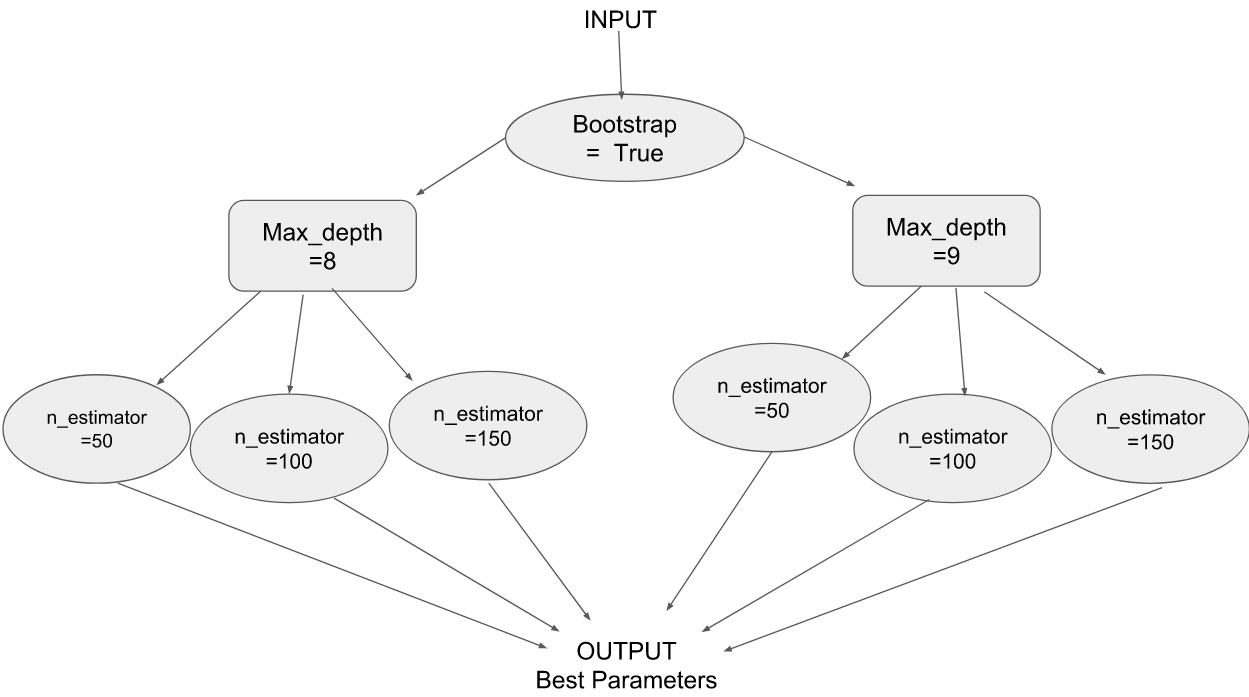## 6. Solving a machine learning problem

We will implement what we have learned so far on the Black Friday dataset and see how it works. Data exploration and treatment is out of the scope of this article as I will only illustrate how to use Dask for a ML problem. In case you are interested in these steps, you can check out the below mentioned articles:

1. Using a simple logistic regression model and making predictions

```#reading the csv files

#having a look at the head of the dataset

#finding the null values in the dataset
df.isnull().sum().compute()

#defining the data and target
categorical_variables = df[['Gender', 'Age', 'Occupation', 'City_Category', 'Stay_In_Current_City_Years', 'Marital_Status']]
target = df['Purchase']

#creating dummies for the categorical variables
data = dd.get_dummies(categorical_variables.categorize()).compute()

#converting dataframe to array
datanew=data.values

#fit the model
lr = LinearRegression()
lr.fit(datanew, target)

```
```#preparing the test data
test_categorical = test[['Gender', 'Age', 'Occupation', 'City_Category', 'Stay_In_Current_City_Years', 'Marital_Status']]
test_dummy = dd.get_dummies(test_categorical.categorize()).compute()
testnew = test_dummy.values

pred=lr.predict(testnew)

```

This will give you the predictions on the given test set.

2. Using grid search and random forest algorithm to find the best set of parameters.

```from dask.distributed import Client
client = Client() # start a local Dask client

from sklearn.externals.joblib import parallel_backend

# Create the parameter grid based on the results of random search
param_grid = {
'bootstrap': [True],
'max_depth': [8, 9],
'max_features': [2, 3],
'min_samples_leaf': [4, 5],
'min_samples_split': [8, 10],
'n_estimators': [100, 200]
}

# Create a based model
from sklearn.ensemble import RandomForestRegressor
rf = RandomForestRegressor()

```
```# Instantiate the grid search model
grid_search = dcv.GridSearchCV(estimator = rf, param_grid = param_grid, cv = 3)
grid_search.fit(data, target)
grid_search.best_params_

```

On printing grid_search.best_params_ you will get the best combination of parameters for the given mode. I have varied only a few parameters here but once you are comfortable with using dask-search, I would suggest experimenting with more parameters while using multiple varying values for each parameter.

```{'bootstrap': True,
'max_depth': 8,
'max_features': 2,
'min_samples_leaf': 5,
'min_samples_split': 8,
'n_estimators': 200}```

One very common question that I have seen while exploring Dask is: How is Dask different from Spark and which one is preferred? There is no hard and fast rule that says one should use Dask (or Spark), but you can make your choice based on the features offered by them and whichever one suits your requirements more.

Here are some important differences between Dask and Spark :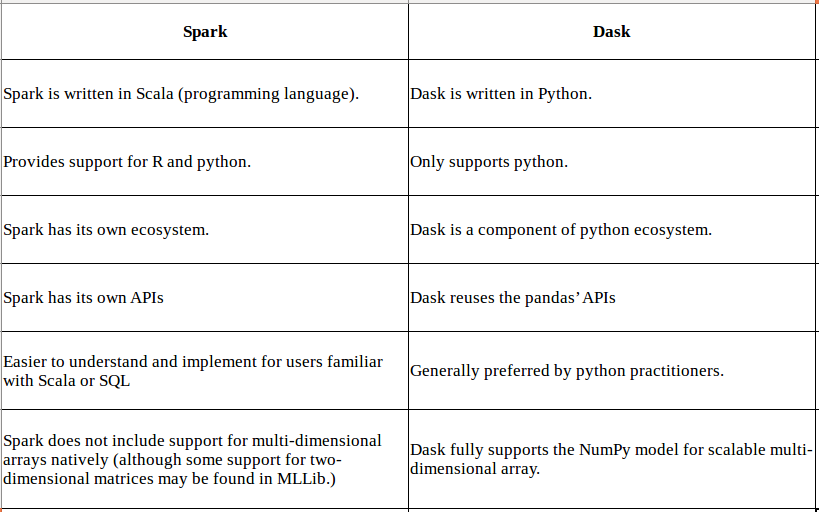## End Notes

I have recently started using Dask and am still exploring this amazing library. It is comforting to know that I don’t have to explore a whole new tool in order to build my models when faced with large datasets. The best part about Dask is that it offers an interface very similar to pandas and there is a very slight (sometimes negligible) difference in the code.

There are innumerable tasks that one can perform using Dask thanks to the drastic reduction in processing time. Go ahead and explore this library and share your experience in the comments section below.###### Aishwarya Singh

An avid reader and blogger who loves exploring the endless world of data science and artificial intelligence. Fascinated by the limitless applications of ML and AI; eager to learn and discover the depths of data science.

## 38 thoughts on "Ultimate guide to handle Big Datasets for Machine Learning using Dask (in Python)"###### Viraj says:August 09, 2018 at 12:38 pm
Thanks for sharing. It sounds like a promising library. Reply###### Nitin says:August 09, 2018 at 3:02 pm
Hello Aishwarya, That's a really awesome utility. Thanks for sharing it. I would like to make an edit in Section 6.2 below *************************************************************** # Instantiate the grid search model grid_search = dcv.GridSearchCV(estimator = rf, param_grid = param_grid, cv = 3) *************************************************************** Here we need to "import dask_searchcv as dcv" to make this command work. And before that one has to install in the env if it's not available. Please update it for the benefit of others. Reply###### Aishwarya Singh says:August 09, 2018 at 3:13 pm
Hi Nitin, Thanks for pointing it out. I missed that line with the code. Have updated the same in the article. Also, the installation steps for dask_searchcv are provided in the previous section. Reply###### Aishwarya Singh says:August 09, 2018 at 5:11 pm###### Jenarthanan says:August 09, 2018 at 8:50 pm
Good article. It would be an added value to the Dask if we added the comparison on runtime stats. Will give a try to use this python package to deal with the huge volume of data! Reply###### Sahar says:August 09, 2018 at 10:07 pm
Thank you very much for sharing this. I can see that Dask has got inherently array or data frame structures, which seems promising, but in terms of performance, how is it comparable with mpi library, which is also used for parallel programming? Reply###### Sandeep Singh says:August 09, 2018 at 11:31 pm
Nice artical, thanks! just a quick one in section "Set up your system: Dask Installation" , we might want to specify how to install it in cluster. Means what are the steps needed to be done for making dask work on more than one machine. Cheers! Reply###### Anshul Saxena says:August 10, 2018 at 10:25 am
Hey, I had a problem executing this statement. Pl see screen shot below: X.chunks AttributeError Traceback (most recent call last) in () 1 #to see the size of each chunk ----> 2 X.chunks AttributeError: 'numpy.ndarray' object has no attribute 'chunks' Reply###### Aishwarya Singh says:August 10, 2018 at 10:51 am
Hi Sahar, Comparing Dask and mpi library in terms of performance, MPI outperforms dask. In fact, Matthew Rocklin has said in an interview, "Dask not going to out-compete MPI on super computers". Reply###### Aishwarya Singh says:August 10, 2018 at 11:01 am###### Aishwarya Singh says:August 10, 2018 at 11:03 am
Hi Anshul, Looks like X in your case is a numpy array. Convert it into a dask array and then execute X.chunks. Reply###### Aishwarya Singh says:August 10, 2018 at 11:05 am
Hi Sandeep, Thanks for the suggestion. Will update it soon. Reply###### Anshul Saxena says:August 10, 2018 at 4:10 pm
i have just copy pasted ur code from section 5.1 till the point where i get this X.chunks error Please elaborate what could be wrong. Reply###### Aishwarya Singh says:August 10, 2018 at 4:52 pm
Hi, Updated the code. Please check now, this should work. import dask.array as da #using arange to create an array with values from 0 to 10 X = da.arange(11, chunks=5) X.compute() #to see size of each chunk X.chunks Reply###### Raymond Doctor says:August 12, 2018 at 8:48 am
Great read. I have parallel data of around 20 lakh strings: English-> Hindi and want to train it on my Windows machine which has 16Gb Ram and a lot of disk space. Any pointers to how to do this. I am new to Python and get lost. Reply###### Adarsh says:August 13, 2018 at 12:58 pm
It's awesome, I hope there won't be any boundary for data size to handle as long as it is less than the size of hard disk (empty space on it). Reply###### Sahar says:August 14, 2018 at 10:30 pm
Thank you for your reply. Does that mean Dask could be preferable because of its simplicity, especially we have a small project? Reply###### Vishal Kumar says:August 15, 2018 at 1:32 pm
Thanks for the article. I would like to ask a question. I am a beginner in Data Science and I am confused to start with pandas or dask. As a beginner which one would be better for me? I have a introductory knowledge of Pandas. I think instead of spending time in pandas, numpy, I should learn Dask instead and get used to it. Reply###### Aishwarya Singh says:August 16, 2018 at 10:22 am
I personally have never worked with text data using dask, but I would suggest you to start with a simpler problem and familiarize yourself with python. If you wish to start with it, first load the dataset and perform basic operations like removing the stop words and punctuations. Reply###### Aishwarya Singh says:August 16, 2018 at 10:26 am
If you are familiar with pandas, learning dask will be extremely simple (it is mostly the same thing). It depends on what kind of data do you come across. If the size of your dataset is not very huge, go for pandas. Reply###### Aishwarya Singh says:August 16, 2018 at 11:25 am
Yes, a python practitioner would certainly prefer dask since the functions are mostly same. Reply###### Rahul says:August 19, 2018 at 5:50 pm
import numpy as np import dask.array as da x = np.arange(1000) #arange is used to create array on values from 0 to 1000 y = da.from_array(x, chunks=(100)) #converting numpy array to dask array y.mean().compute() #computing mean of the array 49.5 Hi Can you please explain how y.mean.compute() is working here is it calculating the mean of only first chunk, if yes then how to get the mean of any i th chunk or of the whole array using using dask Reply###### Aishwarya Singh says:August 20, 2018 at 10:39 am
Hi Rahul, Thanks for pointing that out. If you run the code in the jupyter notebook the result will be 499.5. (updated in the article). Using y.mean.compute() gives the mean of the complete array and not an individual chunk. Reply###### Rahul says:August 22, 2018 at 6:16 pm
Hi Aishwarya, I ran into an error during from dask_ml.import LinearRegression description --- ' --------------------------------------------------------------------------- ContextualVersionConflict Traceback (most recent call last) in () ----> 1 from dask_ml.linear_model import LinearRegression ~\Anaconda3\lib\site-packages\dask_ml\__init__.py in () 2 3 try: ----> 4 __version__ = get_distribution(__name__).version 5 except DistributionNotFound: 6 # package is not installed ~\Anaconda3\lib\site-packages\pkg_resources\__init__.py in get_distribution(dist) 562 dist = Requirement.parse(dist) 563 if isinstance(dist, Requirement): --> 564 dist = get_provider(dist) 565 if not isinstance(dist, Distribution): 566 raise TypeError("Expected string, Requirement, or Distribution", dist) ~\Anaconda3\lib\site-packages\pkg_resources\__init__.py in get_provider(moduleOrReq) 434 """Return an IResourceProvider for the named module or requirement""" 435 if isinstance(moduleOrReq, Requirement): --> 436 return working_set.find(moduleOrReq) or require(str(moduleOrReq)) 437 try: 438 module = sys.modules[moduleOrReq] ~\Anaconda3\lib\site-packages\pkg_resources\__init__.py in require(self, *requirements) 982 included, even if they were already activated in this working set. 983 """ --> 984 needed = self.resolve(parse_requirements(requirements)) 985 986 for dist in needed: ~\Anaconda3\lib\site-packages\pkg_resources\__init__.py in resolve(self, requirements, env, installer, replace_conflicting, extras) 873 # Oops, the "best" so far conflicts with a dependency 874 dependent_req = required_by[req] --> 875 raise VersionConflict(dist, req).with_context(dependent_req) 876 877 # push the new requirements onto the stack ContextualVersionConflict: (dask 0.16.1 (c:\users\acer pc\anaconda3\lib\site-packages), Requirement.parse('dask[array]>=0.18.2'), {'dask-ml'})' Reply###### Aishwarya Singh says:August 23, 2018 at 10:29 am
Hi, Instead of `from dask_ml.import LinearRegression` write `from dask_ml.linear_model import LinearRegression` . Also, please make sure you have performed the installation steps for Dask ML. Reply###### Mallikarjun Bendigeri says:September 10, 2018 at 9:37 am
Hi Aishwaraya, I installed the Dask using the command in my Jupyter. !pip install "dask[complete]" After installation , I getting the below error when I tried to import DataFrame import dask.dataframe as dd Error --------------------------------------------------------------------------- ImportError Traceback (most recent call last) in () 2 import pandas as pd 3 import dask.array as da ----> 4 import dask.dataframe as dd D:\Anaconda\lib\site-packages\dask\dataframe\__init__.py in () 1 from __future__ import print_function, division, absolute_import 2 ----> 3 from .core import (DataFrame, Series, Index, _Frame, map_partitions, 4 repartition, to_delayed) 5 from .io import (from_array, from_pandas, from_bcolz, D:\Anaconda\lib\site-packages\dask\dataframe\core.py in () 29 from ..base import Base, compute, tokenize, normalize_token 30 from ..async import get_sync ---> 31 from . import methods 32 from .utils import (meta_nonempty, make_meta, insert_meta_param_description, 33 raise_on_meta_error) D:\Anaconda\lib\site-packages\dask\dataframe\methods.py in () 5 from toolz import partition 6 ----> 7 from .utils import PANDAS_VERSION 8 9 D:\Anaconda\lib\site-packages\dask\dataframe\utils.py in () 13 import pandas as pd 14 import pandas.util.testing as tm ---> 15 from pandas.core.common import is_datetime64tz_dtype 16 import toolz 17 ImportError: cannot import name 'is_datetime64tz_dtype' Reply###### Aishwarya Singh says:September 10, 2018 at 10:46 am
Hi, The command worked for me. Can you restart the kernel and try again? I checked this issue and apparently restarting the kernel solved the error . If you still face the issue, please let me know. Reply###### Medhy says:September 23, 2018 at 4:33 am
Thanks for this great article. Since I use Dask, I can't change for pyspark, this tool is awesome. But Today I have a problem, I've got a : ModuleNotFoundError: No module named 'dask_searchcv' And My installation of dask is good. When I do pip install dask-searchcv, I have a Requirement already satisfied. So I dont kwow what to do. Reply###### Aishwarya Singh says:October 17, 2018 at 4:47 pm
Hi Medhy, Did you use pip install or conda install? Reply###### Arman says:October 23, 2018 at 2:30 am###### Aishwarya Singh says:October 23, 2018 at 10:25 am
Hi, Thanks for this suggestion Arman. For now you can bookmark the articles. Reply###### NC says:October 23, 2018 at 10:34 pm
I am actually finding difficult for a use case where Dask will be faster than Pandas. Your example of read_csv is not true because you did not compute, thus it reads nothing from the csv. Reply###### Aishwarya Singh says:October 24, 2018 at 11:12 am
Hi NC, When I started with it, I had the same doubt; try implementing a model on a dataset that's larger than the RAM on your system using pandas and DASK. Reply###### Supriya says:October 29, 2018 at 1:34 pm
I have a use case where my file size may vary upto 10GB. I tired to use pandas and failed to process validations due to memory constraint, And now I went through pyspark dataframe sql engine to parse and execute some sql like statement in in-memory to validate before getting into database. Does pyspark sql engine reliable? Or is there any way to do it using pandas or any other modules. I see using spark for small set of data id not recommended. I am entirely new to python. Please help me understand and fit my use case. Reply###### Aishwarya Singh says:October 31, 2018 at 12:16 pm
Hi Supriya, I haven't worked with spark so far but here are a few blogs you can refer. Hope it helps! Reply###### Nick says:December 15, 2018 at 5:55 pm
Hi AS, The array testnew that is created from the dataframe data when get_dummies() is applied, is a numpy array since you used the compute() method...right? Wouldn't be better if a dask array (or dataframe was used instead)? Reply###### Reaz says:January 17, 2019 at 6:32 pm
AISHWARYA, thank you for sharing this great article. Reply